Question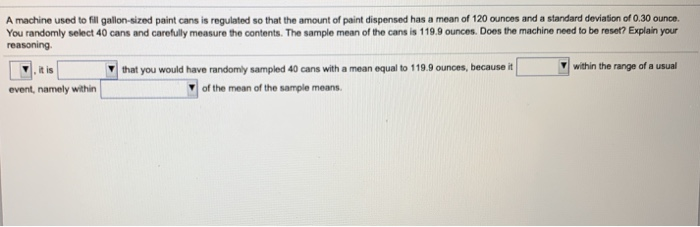Given that

The standard error of mean is

The usual range of values is within 2 standard deviations of mean. Here usual range of values will be

Yes.It is unusual that you would have randomly sampled 40 cans with a mean equal to 119.9 ounces because it is not within range of a usual event namely with 2 standard deviations of the mean of the sample means.

#### Earn Coins

Coins can be redeemed for fabulous gifts.

Similar Homework Help Questions
• ### core: 0 of 2 pts .4.35 A machine used to fil galon-sized paint cans is reguiated...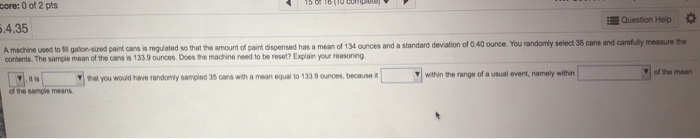core: 0 of 2 pts .4.35 A machine used to fil galon-sized paint cans is reguiated Question Hoip so that the amount of paint dispensed has a mean of 134 ounces and a standard deviation of 0.40 ounce. You randomly select 35 cans and carefully measure the contents.The sample mean of the cans is 1339 ounces. Does the machine need to be reset? Explain your reasoning that you would have randomly sampled 35 cans with a mean equal to 1330ounoes,...

• ### first drop down is yes or no second is likely or very unlikely third is lies...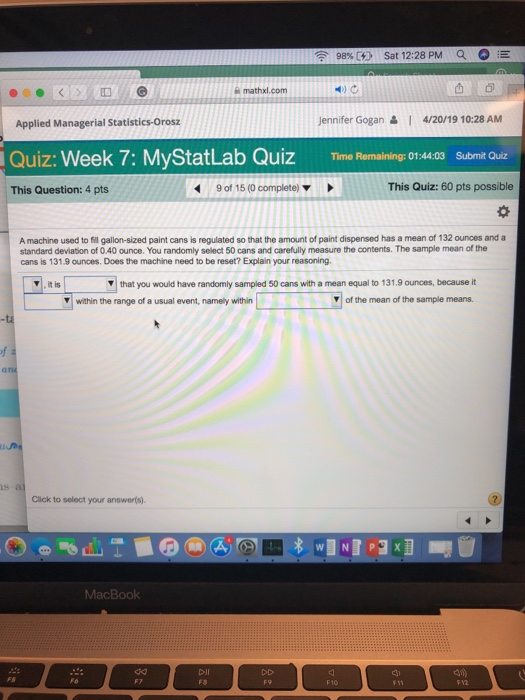first drop down is yes or no second is likely or very unlikely third is lies or does not lie fourth is within 1 standard deviation, 2, or 3 98% Sat 12:28 PM a ! mathxl.com Jennifer Gogan &1 4/20/19 10:28 AM Applied Managerial Statistics-Orosz Quiz: Week 7: MyStatLab Quiz ThmRemaining 01:44.09 SubmitQ This Quiz: 60 pts possible 9 of 15 (0 complete) This Question: 4 pts A machine used to fill gallon-sized paint cans is regulated so that the...

• ### 5. Problem 5: A bottling machine can be regulated so that it discharges an average of...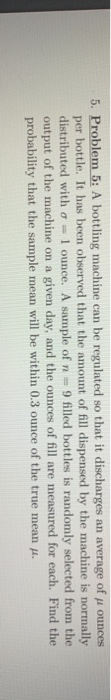5. Problem 5: A bottling machine can be regulated so that it discharges an average of pi ounces per bottle. It has been observed that the amount of fill dispensed by the machine is normally distributed with a = 1 ounce. A sample of n = 9 filled bottles is randomly selected from the output of the machine on a given day, and the ounces of fill are measured for each. Find the probability that the sample mean will be...

• ### A paint manufacturer uses a machine toll gallon cars with paint (1 128 ounces). The manufacturer...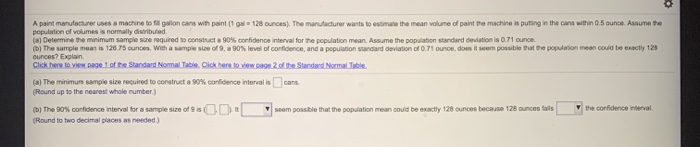A paint manufacturer uses a machine toll gallon cars with paint (1 128 ounces). The manufacturer wants to estimate the mean volume of paint the machine is puting in the card within 0.5 ounce Assume the population of volumes is normally distribuid. (8) Determine the minimum sample size required to constructa 90% confidence interval for the population mean. Assume the population standard deviation is 0.71 ounce (0) The sample meanis 126.75 ounces with a sample size of 9, a 90%...

• ### A machine set to fill soup cans with a mean of 20 ounces and a standard...

A machine set to fill soup cans with a mean of 20 ounces and a standard deviation of 0.1 ounces. A random sample of 34 cans has a mean of 20.02 ounces. Should the machine be reset? No, the probability of this outcome at 0.122, would be considered usual, so there is no problem No the probability of this outcome at 0.421 would be considered usual, so there is no problem Yes, the probability of this outcome at 0.878 would...

• ### A machine used to fill cans of Campbell’s tomato soup (low salt) has the following characteristics:...

A machine used to fill cans of Campbell’s tomato soup (low salt) has the following characteristics: µ = 12 ounces and s = .5 ounces. a. Depict graphically the sampling distribution of all possible values of , where is the sample mean (point estimator) for 30 cans selected randomly by a quality control inspector. b. What is the probability of selecting a sample of 36 cans with a sample mean greater than 12.2 ounces?

• ### Orange 52. Juice Dispensing Machine A beverage company uses a machine to fill half-gallon bottles with...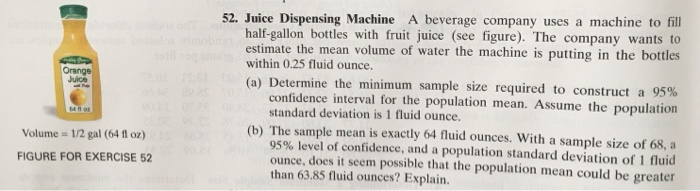Orange 52. Juice Dispensing Machine A beverage company uses a machine to fill half-gallon bottles with fruit juice (see figure). The company wants to estimate the mean volume of water the machine is putting in the bottles within 0.25 fluid Ounce. (a) Determine the minimum sample size required to construct a 95% confidence interval for the population mean. Assume the population standard deviation is 1 fluid Ounce. (b) The sample mean is exactly 64 fluid ounces. With a sample size...

• ### The amount of corn chips dispensed into a 13-ounce bag by the dispensing machine has been identified as possessing a normal distribution with a mean of 13.5 ounces and a standard deviation of 0.3 ounce. Suppose 40 bags of chips were randomly selected from

The amount of corn chips dispensed into a 13-ounce bag by the dispensing machine has been identified as possessing a normal distribution with a mean of 13.5 ounces and a standard deviation of 0.3 ounce. Suppose 40 bags of chips were randomly selected from this dispensing machine. Find the probability that the sample mean weight of these 40 bags exceeded 13.6 ounces.

• ### Sampling Distributions 1. An automatic machine used to ill cans of soup has a mean flling...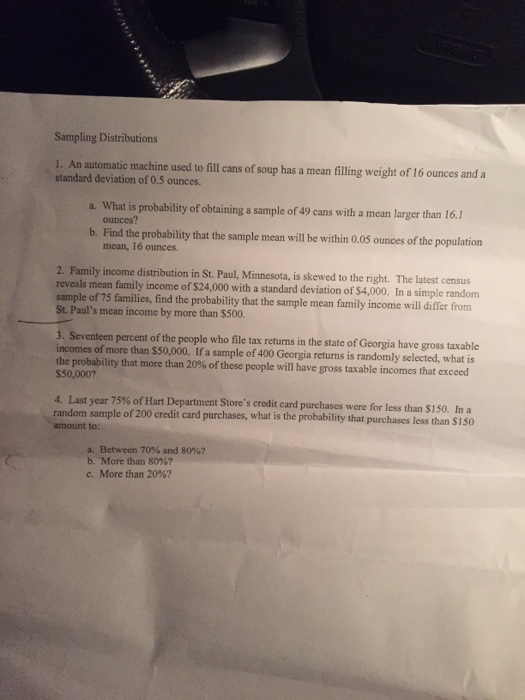Sampling Distributions 1. An automatic machine used to ill cans of soup has a mean flling weight of 16 ounces and a standard deviation of 0.5 ounces. a. What is probability of obtaining a sample of 49 cans with a mean larger than 16.1 b. Find the probability that the sample mean will be within 005 ounces of the population ounces? mean, 16 ounces. 2. Family income distribution in St. Paul, Minnesota, is skewed to the right. The latest census...

• ### Question 1 The average math SAT score is 511with a standard deviation of 119. A particular...

Question 1 The average math SAT score is 511with a standard deviation of 119. A particular high school claims that its students have unusually high math SAT scores. A random sample of 50 students from this school was​ selected, and the mean math SAT score was 555. Is the high school justified in its​ claim? Explain. ▼ Pick one No Yes ​because the​ z-score (what is the z score) ​(?​) is ▼ pick one not unusual unusual since it ▼...